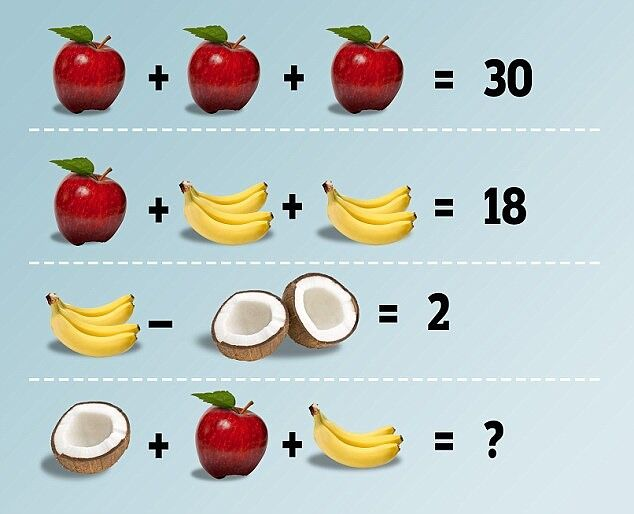Voomed

# This Fruit Based Puzzle Is Dividing Opinion And Driving Facebook Mad On What The Right Answer Is

OK prepare for opinion to be divided. This isn't quite at the standard of the now infamous blue-black/white-gold dress that shook the world to its very core about a year ago, but it's still an intriguing puzzler. It involves a picture of various equations where a different fruit amounts to a certain number.

And that's where the problem lies. And it's driving some people on Facebook mad with rage over the different ways to work out the answer to the problem. Let's explain. In the picture (below) the first equation shows three apples equaling the figure 30.

So, therefore, each apple must equal 10.

The second line features an apple and two bunches of four bananas. Together they add up to 18, so apple is 10 the bananas must equal four each.

Third line, bunch of bananas minus two coconuts equals two. So, therefore, if bananas equal four then the coconuts must equal two. Are you still with us. All pretty standard math so far, right?

The fourth line is where things get controversial. It shows ONE coconut plus one apple plus THREE bananas. And then a question mark as to what they add up to.

So what do you think the answer is?Some people think it's 14, because an apple is 10, four bananas is four so three must be three, and two coconuts is two so one must be one. So it would be 10 + 3 + 1 = 14.

The other camp is that no matter how many bananas or coconuts there are bananas always equal four and coconuts always equal two. So it would be 10 + 4 + 2 = 16.

If you think you know the right answer, then let Dr Kevin Bowman a mathematician from the University of Central Lancashire, England, throw you. Because he told the Daily Mail this about the puzzle:

You can interpret it in many ways; one way is no more correct than another. There’s no ambiguity in the first equation; 3 apples is 30, so one apple is worth 10. But because all the bananas aren’t the same, you could say that they all represent different amounts. You might even say that the two coconut pieces in the third equation are different sizes, and therefore add up to three quarters or even seven eighths when put together. In that sense, there are an infinite amount of possible answers.

So there you go, the correct answer is: infinity. Or something.